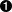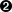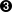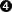# Creating Custom Java Functions

You can call custom Java functions directly in StreamBase expressions by an alias defined in the server configuration file, or by using the `calljava()` function. The StreamBase expression language provides two forms of the `calljava()` function, to be used in StreamBase simple and aggregate expressions.

This topic provides guidelines for creating StreamBase custom functions in Java.

## Custom Java Function Samples

The StreamBase installation includes the source files for two custom Java function samples:

Sample Described in
custom-java-function Custom Java Simple Function Sample
custom-java-aggregate Custom Java Aggregate Function Sample

Custom aggregate functions are built by extending the `com.streambase.sb.operator.AggregateWindow` class of the StreamBase Java Client library. This class is described in Java API Documentation.

## Creating Custom Java Simple Functions

Simple custom Java functions can be called in expressions in the context of most StreamBase components, other than the Aggregate operator. Follow these steps to create a custom Java simple function:

For example, `Hypotenuse.java` in the sample application declares the following class and method:

```public class Hypotenuse {

public static double calculate(double a, double b) {
return Math.sqrt(a*a + b*b);
}
}```

At compile time, the `calljava()` implementation looks for only a single method matching the exact types of the function call in the StreamBase expression. But there can be multiple matching methods, such as these two functionally equivalent ones:

```public static boolean isZero(int i) { return i == 0; }
public static Boolean isZero(Integer i) {
return i == null ? null : Boolean.valueOf(i.intValue() == 0);
}```

If this case occurs, StreamBase throws an error.

## Creating Custom Java Aggregate Functions

Follow these steps to create a custom Java aggregate function:

Consider the following annotated example:

```package com.mycompany;

import com.streambase.sb.operator.AggregateWindow;

public class MyStdev extends AggregateWindow {private double sum;
private double sumOfSquares;
private int count;

public void init() {sum = sumOfSquares = 0.0;
count = 0;
}

public double calculate() {return Math.sqrt((count * sumOfSquares - sum*sum) / count*(count-1));
}

public void accumulate(double value) {sum += value;
sumOfSquares += value*value;
++count;
}

public void release() { /* nothing to release in this example */ }}```

The following annotations describe points of interest in the preceding example:

 Declare a public class that extends the `AggregateWindow` class (as documented in the StreamBase Java Client library). The `init()` method is called at the start of each new use of the class. Since custom aggregate objects are likely to be reused, perform all initialization in `init()` rather than in the constructor. (The constructor is called only once, while `init()` is called before each use.) Your implementation must contain a `calculate()` method that takes no arguments and returns a value that is convertible to a StreamBase data type. The `calculate()` method may be called several times, or not at all. Your implementation must provide at least one `accumulate()` method, and can optionally provide several overloaded `accumulate()` methods, one per data type. `calljava()` determines which one to call based on type. The argument types for `accumulate()` and the return type for `calculate()` can be any of the types described in the table in the next section. The `release()` method is called at the end of each use of the class.

## Method Parameter and Return Types

The method can have any number of parameters, including none. Each parameter must be a Java primitive or object type corresponding to a StreamBase data type as shown in the following table:

StreamBase Data Type Java Primitive Java Object
blob com.streambase.sb.ByteArrayView
bool boolean java.lang.Boolean
double double java.lang.Double
int int java.lang.Integer
long long java.lang.Long
list primitive_type[] java.util.List
string byte[] java.lang.String
timestamp com.streambase.sb.Timestamp
tuple com.streambase.sb.Tuple

Notes

• For simple functions, the return type cannot be void, and must be one of the Java primitive or Java Object types shown above.

• You can use `java.lang.String` anywhere a byte[] is acceptable as an argument or return value. In this case, the StreamBase string is transparently converted to or from a `java.lang.String` using the system default encoding.

• If a parameter's type is byte[] and its value is null, it is represented as a Java `null`. Likewise, if a Java method with a byte[] return type returns a null, the calling StreamBase expression will see the return value as string(null).

• You can pass a list of lists to functions expecting multi-dimensional array arguments. You can mix list and array notations when doing this. That is, a two-dimensional list of doubles can be passed to functions accepting any of the following arguments:

• `double[][]`

• `list<double[]>`

• `list<list<double>>`

### Note

Only Java primitive arrays and string arrays are compatible with lists, not complex data types such as timestamps or tuples. Data type coercion is not supported.

### Note

When an array argument has more than two dimensions, you cannot use a function alias. You must invoke the function via `calljava()`.

• For better performance, Java functions can return arrays in place of lists. For example, the following return types are equivalent:

```double[]     foo() {}   // == list(double)
double[][][] foo() {}   // == list(list(list(double)))```
• If the parameter or return type is list or tuple, you must either provide a custom function resolver, or must define the argument types in a `<custom-function>` element in the server configuration file. See Custom Functions with Complex Data Types for details.

• If the value of a parameter with a primitive type is null at run time, the method that implements the custom function is invoked and returns a null result and a console warning is then issued. You can also cause an error tuple to be emitted on the operator's error port (if any). To do so, you must set the following system property, which by default is `false`, to `true`, as follows:

`-Dstreambase.codegen.error-for-null-as-primitive=true`

For example, if a StreamBase custom function call would involve converting a StreamBase `int(null)` or `bool(null)` value to a primitive Java `int` or `boolean`, the method is called, and returns a null value.

```public static boolean isZero(int i) { return i == 0; }

calljava("TheClass", "isZero", 1)          /* false *
calljava("TheClass", "isZero", 0)          /* true */
calljava("TheClass", "isZero", int(null))  /* null */```

If you have enabled emission of error tuples as described above, their content will be similar to the following:

```{'description':'Evaluation exception: in file `<name>`.sbapp element `<operator>`,
at expression "calljava(\'test.WithNull\', \'WithNull\', input1.x)",
nulls are passed as arguments to function WithNull which expects primitive types.
The result of this function call will be null', 'operatorName':'`<name>`',
'inputPort':0,'nodeName':'`<name>`','type':'eval-error','action':'continue',
'time':'`<timestamp>`'}```